# Prime number

2010 Mathematics Subject Classification: Primary: 11A41 [MSN][ZBL]

A natural number (positive integer)that has only the two divisors 1 and. E.g.,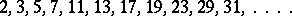Natural numbers which are not prime are called composite. The concept of a prime number is fundamental in the study of divisibility of natural numbers. Thus, the fundamental theorem of elementary number theory states that every natural number, different from one, is either prime or, if it is composite, can be represented by a product of prime numbers. This representation is, moreover, unique (up to the order of the factors). A description of this decomposition in the form of powers of identical prime numbers and in increasing order is given by the canonical decomposition of a natural number: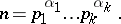By using the canonical decompositions of natural numbers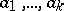one can find their greatest common divisor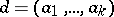and least common multiple. By means of the canonical decomposition of a natural numberone can compute the values of the number-theoretic functions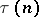,and, which denote, respectively, the number of divisors of, the sum of the divisors ofand the amount of natural numbersthat are coprime with(i.e. are such that):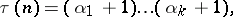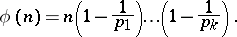An essential feature of these formulas is their dependence on the arithmetical structure of.

The prime numbers play the role of "construction blocks" from which one can construct all other natural numbers. Already in the 3rd century B.C., Euclid proved that the set of prime numbers is infinite, and Eratosthenes found a way of sifting the prime numbers out of the sequence of natural numbers (cf. Eratosthenes, sieve of). L. Euler found a proof of the infinity of the set of prime numbers based on the use of tools of mathematical analysis. The further development of Euler's analytic method proved to be fruitful (cf. Analytic number theory). P.L. Chebyshev discovered new laws to which prime numbers are subject. In particular, using the canonical decomposition of the number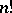he found an inequality for the amountof prime numbers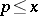:where,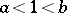, are certain positive constants. The most refined laws to which the behaviour of the sequence of prime numbers is subject were obtained by refining the original ideas of Chebyshev and by using analytic and, in a number of cases, elementary methods (cf. Distribution of prime numbers).

Prime numbers are related not only to the multiplicative structure but also to the additive structure of natural numbers. Goldbach's problem on the partitioning of a natural number into a sum of three prime numbers is characteristic in this respect (cf. Goldbach problem). It was solved in 1937 by I.M. Vinogradov (cf. Additive number theory). The study of laws of decomposing prime numbers in algebraic fields shed light on properties of ordinary prime numbers. E.g., by considering the decompositions of prime numbers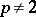in the Gaussian number field (cf. Gauss number) one obtains Euler's theorem:if and only if(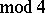).

Up till now (1990) there are a number of unsolved problems related to prime numbers. E.g.,

Is the set of Mersenne prime numbers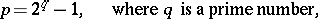infinite?

Is the set of Fermat prime numbers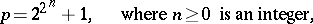infinite?

Does there exist an infinite set of prime "twins", i.e. prime numbers such that?

Experimental and heuristic considerations favour positive answers to the questions stated above, as well as to a number of similar questions.